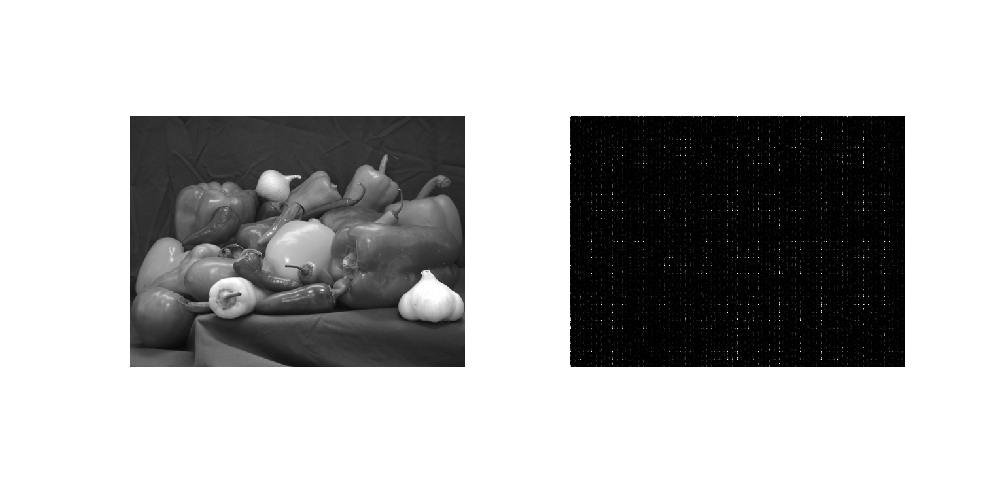• 哈达玛变换本质上是一种特殊排序的沃尔什变换，哈达玛变换矩阵也是一个方阵，只包括+1和-1两个矩阵元素，各行或各列之间彼此是正交的。哈达玛变换矩阵具有简单的递推关系，即高阶矩阵可用两个低阶矩阵求得。这个特点...图像处理
• 由于沃尔什函数是二值正交函数，与数字逻辑中的两个状态相对应，因此它更适用于计算机技术、数字信号处理一维沃尔什变换N=2nN=2^nN=2n哈达玛变换与沃尔什变换类似，由哈达玛变换核组成的矩阵是一个正交对称矩阵，...
沃尔什变换
沃尔什变换是由+1或-1的基本函数的级数展开而成的，满足完备正交特性，属于方波型正交变换。由于沃尔什函数是二值正交函数，与数字逻辑中的两个状态相对应，因此它更适用于计算机技术、数字信号处理
一维沃尔什变换
沃尔什变换要求空域矩阵阶数$N$满足$N=2^n$，若满足，则有：$F(\mu)=\sum_{x=0}^{N-1}f(x)g(x,\mu)$$g(x,\mu)=\frac{1}{N}\prod_{i=0}^{n-1}(-1)^{\sum_{i=0}^{n-1}b_i(x)b_{n-1}(\mu)}$一维沃尔什反变换：$f(x)=\sum_{x=0}^{N-1}F(\mu)h(x,\mu)$$h(x,\mu)=\prod_{i=0}^{n-1}(-1)^{\sum_{i=0}^{n-1}b_i(x)b_{n-1}(\mu)}$其中，$b_i(x)$是$x$的二进制表达式中第$i$位的意思
例如$N=2^n$，$n=3$，$x=6$，则有$x=6=(110)_2$，可得$b_0(x)=0$、$b_1(x)=1$、$b_2(x)=1$
与傅立叶变换不同，沃尔什变换的变换核是由+1或-1构成的二值对称矩阵
例如：求$N=2^2$的沃尔什变换$F(0)=\frac{1}{4}\sum_{x=0}^{3}[f(x)\prod_{i=0}^{1}(-1)^{b_i(x)+b_{1-i}(0)}]=\frac{1}{4}[f(0)+f(1)+f(2)+f(3)]$$F(1)=\frac{1}{4}\sum_{x=0}^{3}[f(x)\prod_{i=0}^{1}(-1)^{b_i(x)+b_{1-i}(1)}]=\frac{1}{4}[f(0)+f(1)-f(2)-f(3)]$$F(2)=\frac{1}{4}\sum_{x=0}^{3}[f(x)\prod_{i=0}^{1}(-1)^{b_i(x)+b_{1-i}(2)}]=\frac{1}{4}[f(0)-f(1)+f(2)-f(3)]$$F(3)=\frac{1}{4}\sum_{x=0}^{3}[f(x)\prod_{i=0}^{1}(-1)^{b_i(x)+b_{1-i}(3)}]=\frac{1}{4}[f(0)-f(1)-f(2)+f(3)]$其中方括号中各项的符号即位变换核的符号
可见沃尔什变换的本质是将离散序列$f(x)$中各项值的符号按规律改变进行加减运算
二维沃尔什变换
$F(\mu,\nu)=\frac{1}{N}\sum_{x=0}^{N-1}\sum_{x=0}^{N-1}f(x,y)g(x,y,\mu,\nu)$$f(x,y)=\frac{1}{N}\sum_{\mu=0}^{N-1}\sum_{\nu=0}^{N-1}F(\mu,\nu)g(x,y,\mu,\nu)$$g(x,y,\mu,\nu)=G=\frac{1}{N}\prod_{i=0}^{n-1}(-1)^{\sum_{i=0}^{n-1}[b_i(x)b_{n-1-i}(\mu)+b_i(y)b_{n-1-i}(\nu)]}$表达为矩阵形式为$F=\frac{1}{N^2}GfG$$f=GFG$
与傅立叶变换一样，二维沃尔什变换是可分离的，可以通过两个一维沃尔什变换完成计算$g(x,y,\mu,\nu)=g_1(x,\mu)g_2(y,\nu)$
实例：求离散沃尔什变换
$f=\begin{bmatrix} 1 & 3 & 3 & 1 \\ 1 & 3 & 3 & 1 \\ 1 & 3 & 3 & 1 \\ 1 & 3 & 3 & 1 \\ \end{bmatrix}$可知$N=4$，其变换核为$G=\begin{bmatrix} 1 & 1 & 1 & 1 \\ 1 & 1 & -1 & -1 \\ 1 & -1 & 1 & -1 \\ 1 & -1 & -1 & 1 \\ \end{bmatrix}$则有$F=\frac{1}{N^2}GfG=\frac{1}{4^2}\begin{bmatrix} 1 & 1 & 1 & 1 \\ 1 & 1 & -1 & -1 \\ 1 & -1 & 1 & -1 \\ 1 & -1 & -1 & 1 \\ \end{bmatrix}\begin{bmatrix} 1 & 3 & 3 & 1 \\ 1 & 3 & 3 & 1 \\ 1 & 3 & 3 & 1 \\ 1 & 3 & 3 & 1 \\ \end{bmatrix}\begin{bmatrix} 1 & 1 & 1 & 1 \\ 1 & 1 & -1 & -1 \\ 1 & -1 & 1 & -1 \\ 1 & -1 & -1 & 1 \\ \end{bmatrix}=\begin{bmatrix} 2 & 0 & 0 & -1 \\ 0 & 0 & 0 & 0 \\ 0 & 0 & 0 & 0 \\ 0 & 0 & 0 & 0 \\ \end{bmatrix}$可以看出，沃尔什变换具有信息集中的作用，原始数据中数字越是均匀分布，变换后的数据越是集中于边角区域
哈达玛变换
与沃尔什变换类似，由哈达玛变换核组成的矩阵是一个正交对称矩阵，属于方波型正交变换，不同之处在于其行、列次序不一样。
一维哈达玛变换
哈达玛变换要求空域矩阵阶数$N$满足$N=2^n$，若满足，则有：$F(\mu)=\sum_{x=0}^{N-1}f(x)g(x,\mu)$$g(x,\mu)=\frac{1}{N}(-1)^{\sum_{i=0}^{n-1}b_i(x)b_{i}(\mu)}$一维哈达玛反变换：$f(x)=\sum_{x=0}^{N-1}F(\mu)h(x,\mu)$$h(x,\mu)=(-1)^{\sum_{i=0}^{n-1}b_i(x)b_{i}(\mu)}$$b_i(x)$是$x$的二进制表达式中第$i$位的意思
二维哈达玛变换
$F(\mu,\nu)=\frac{1}{N}\sum_{x=0}^{N-1}\sum_{x=0}^{N-1}f(x,y)g(x,y,\mu,\nu)$$f(x,y)=\frac{1}{N}\sum_{\mu=0}^{N-1}\sum_{\nu=0}^{N-1}F(\mu,\nu)g(x,y,\mu,\nu)$$g(x,y,\mu,\nu)=G=\frac{1}{N}(-1)^{\sum_{i=0}^{n-1}[b_i(x)b_{i}(\mu)+b_i(y)b_{i}(\nu)]}$二维哈达玛变换是可分离的，可变换为两个一维哈达玛变换来进行计算。
列率
在哈达玛矩阵中，沿列方向上符号改变的次数称为列率，类似傅立叶变换中频率的概念。
例如有矩阵$H=\begin{bmatrix} 1 & 1 & 1 & 1 \\ 1 & -1 & 1 & -1\\ 1 & 1 & -1 & -1 \\ 1 & -1 & -1 & 1\\ \end{bmatrix}$
其第一列元素符号无变化，于是第一列的列率为0；
第二列$a_{12}$到$a_{22}$符号由正变负、$a_{22}$到$a_{32}$符号由负变正、$a_{32}$到$a_{42}$符号由正变负，共改变3次，因此第二列的列率为3；以此类推，第三列的列率为1，第四列的列率为2
哈达玛变换在列率上是随机的，不利于实现逐次倍加法的快速运算，但是能导出一个简单的递推关系，构造其变换矩阵，而后进行哈达玛正向和反向变换
哈达玛变换核矩阵
在满足$N=2^n$的情况下
有最低阶$N=2$时的哈达玛变换矩阵$H_2$为：$H_2=\begin{bmatrix} 1 & 1 \\ 1 & -1 \\ \end{bmatrix}$有任意阶$N=2N$时的哈达玛矩阵为：$H_{2N}=\begin{bmatrix} H_N & H_N \\ H_N & -H_N \\ \end{bmatrix}$由此可推得：$H_{4}=\begin{bmatrix} H_2 & H_2 \\ H_2 & -H_2 \\ \end{bmatrix},H_{8}=\begin{bmatrix} H_4 & H_4 \\ H_4 & -H_4 \\ \end{bmatrix},...$
将哈达玛矩阵按照列率顺序排列，得到定序的哈达玛变换对为：$F(\mu,\nu)=\frac{1}{N}\sum_{x=0}^{N-1}\sum_{x=0}^{N-1}f(x,y)g(x,y,\mu,\nu)$$f(x,y)=\frac{1}{N}\sum_{\mu=0}^{N-1}\sum_{\nu=0}^{N-1}F(\mu,\nu)g(x,y,\mu,\nu)$$g(x,y,\mu,\nu)=G=\frac{1}{N}(-1)^{\sum_{i=0}^{n-1}[b_i(x)p_{i}(\mu)+b_i(y)p_{i}(\nu)]}$其中\begin{aligned} p_0(\mu) & = b_{n-1}(\mu) \\ p_i(\mu) & = b_{n-i}(\mu)+b_{n-1-i}(\mu) \\ \end{aligned}表达为矩阵形式为$F=\frac{1}{N^2}HfH$$f=HFH$
实例：求离散哈达玛变换$f=\begin{bmatrix} 1 & 3 & 3 & 1 \\ 1 & 3 & 3 & 1 \\ 1 & 3 & 3 & 1 \\ 1 & 3 & 3 & 1 \\ \end{bmatrix}$易知$N=4$，其哈达玛变换核矩阵为：$H_{4}=\begin{bmatrix} H_2 & H_2 \\ H_2 & -H_2 \\ \end{bmatrix}=\begin{bmatrix} 1 & 1 & 1 & 1 \\ 1 & -1 & 1 & -1\\ 1 & 1 & -1 & -1 \\ 1 & -1 & -1 & 1\\ \end{bmatrix}$$H_4$按列率排序后得到$H_4=\begin{bmatrix} 1 & 1 & 1 & 1 \\ 1 & 1 & -1 & -1\\ 1 & -1 & -1 & 1 \\ 1 & -1 & 1 & -1\\ \end{bmatrix}$由此按照哈达玛变换的计算公式可得$F=\frac{1}{N^2}H_4fH_4=\begin{bmatrix} 2 & 0 & -1 & 0 \\ 0 & 0 & 0 & 0\\ 0 & 0 & 0 & 0 \\ 0 & 0 & 0 & 0\\ \end{bmatrix}$
将沃尔什变换与哈达玛变换进行对比可以发现，两个变换的结果之间只是矩阵列的顺序不同，不会影响两者应用于压缩时的效率


展开全文沃尔什变换 正交变换 图像处理
• 沃尔什-哈达玛变换（Walsh-Hadmard ...    哈达玛变换是利用哈达玛矩阵作为变换矩阵新实施的遥感多光谱域变换。哈达玛矩阵为一个对称的正交矩阵。哈达变换实际是将坐标轴旋转45°的正交变换。     沃尔什-哈
沃尔什-哈达玛变换（Walsh-Hadmard Transform,WHT）

沃尔什-哈达玛变换（Walsh-Hadmard Transform,WHT）

1. 前言
2.数学表达式

2.1 一维哈达玛正变换及反变换
2.2 二维哈达玛正变换及反变换
2.3 沃尔什变换
2.4 沃尔什离散变换

3. 代码实现

1. 前言
哈达玛变换是利用哈达玛矩阵作为变换矩阵新实施的遥感多光谱域变换。哈达玛矩阵为一个对称的正交矩阵。哈达变换实际是将坐标轴旋转45°的正交变换。
沃尔什-哈达玛变换（Walsh-Hadmard Transform,WHT），是一种典型的非正弦函数变换，采用正交直角函数作为基函数，具有与傅里叶函数类似的性质，图像数据越是均匀分布，经过沃尔什-哈达玛变换后的数据越是集中于矩阵的边角上，因此沃尔什变换具有能量集中的性质，可以用于压缩图像信息。
2.数学表达式

哈达玛矩阵：哈达玛矩阵的元素由+1和-1组成的下交方阵
哈达玛变换要求图像的大小为$N=2^n$

2.1 一维哈达玛正变换及反变换
正换公式如下：
$H(u) = \frac{1}{n}\sum_{x=0}^{n-1}{f(x)(-1)^{\sum_{i=0}^{n-1}{b_i(x)b_i(u)}}}$
反变换公式如下：
$f(x) = \sum_{u=0}^{n-1}{H(u)(-1)^{\sum_{i=0}^{n-1}{b_i(x)b_i(u)}}}$
2.2 二维哈达玛正变换及反变换
正换公式如下：
$H(u,v) = \frac{1}{N}\sum_{x=0}^{N-1}\sum_{y=0}^{N-1}{f(x,y)(-1)^{\sum_{i=0}^{n-1}{[b_i(x)b_i(u)+b_i(y)b_i(v)]}}}$
反变换公式如下：
$f(x,y) = \frac{1}{N}\sum_{u=0}^{N-1}\sum_{v=0}^{N-1}{H(u,v)(-1)^{\sum_{i=0}^{n-1}{[b_i(x)b_i(u)+b_i(y)b_i(v)]}}}$
2.3 沃尔什变换
由哈达玛的表达式可以知道，哈达玛变换矩阵的列率的排列是无序的，如果将哈达玛核进行列率的排序，即得到的有序的变换就是沃尔什(Walsh)变换。
一维Walsh变换核为：
$g(x,u) = \frac{1}{N}\prod_{i=0}^{N-1}{(-1)^{\sum_{i=0}^{n-1}b_i(x)b_{n-1-i}(u)}}$
二维沃尔什正变换
$W(u,v) = \frac{1}{N}\sum_{x=0}^{N-1}\sum_{y=0}^{N-1}{f(x,y)\prod_{i=0}^{n-1}(-1)^{\sum_{i=0}^{n-1}{[b_i(x)b_{n-1-i}(u)+b_i(y)b_{n-1-i}(v)]}}}$
二维沃尔什反变换
$f(x,y) = \frac{1}{N}\sum_{x=0}^{N-1}\sum_{y=0}^{N-1}{W(u,v)\prod_{i=0}^{n-1}(-1)^{\sum_{i=0}^{n-1}{[b_i(x)b_{n-1-i}(u)+b_i(y)b_{n-1-i}(v)]}}}$
2.4 沃尔什离散变换
一维离散沃尔什正变换
$W(u) = \frac{1}{N}\sum_{x=0}^{N-1}{f(x)Wal_H(u,x)}$
一维离散沃尔什反变换
$f(x) = \frac{1}{N}\sum_{x=0}^{N-1}{W(u)Wal_H(u,x)}$
二维离散沃尔什正变换
$W(u,v) = \frac{1}{MN}\sum_{x=0}^{M-1}\sum_{y=0}^{N-1}{f(x,y)Wal_H(u,v)Wsl_H(v,y)}$
二维离散沃尔什反变换
$f(x,y) = \frac{1}{MN}\sum_{x=0}^{M-1}\sum_{y=0}^{N-1}{W(u,v)Wal_H(u,v)Wsl_H(v,y)}$
其中：$x,u=0,1,2,...,M-1;y,v=0,1,2,...N-1$
3. 代码实现

import scipy
from scipy.linalg import hadamard
import cv2
import numpy as np

# 注意图像大小一定是2的幂次方
src = cv2.imread('resources/images/lena.png',0)
srcf = np.float32(src)
h1,h2 = src.shape

# 生成哈达玛变换矩阵
H1 = hadamard(h1)
H2 = hadamard(h2)

# 哈达玛变换
HJ = H1.dot(srcf).dot(H2)
J = HJ / np.sqrt(h1*h2)

# 哈达玛变换逆变换
RJ = H1.T.dot(J).dot(H2.T)
HRJ = RJ / np.sqrt(h1*h2)

J = np.clip(J,0,255).astype(np.uint8)
HRJ = np.clip(HRJ,0,255).astype(np.uint8)

cv2.imshow(u'Source',src)
cv2.imshow(u'Hadamard Transform Result',J)
cv2.imshow(u'hadamard Transform Inverse Result',HRJ)
cv2.waitKey()
cv2.destroyAllWindows()

程序运行结果：第一张图像为原图像，第二张为哈达玛变换结棍，第三张为哈达玛反变换结果


展开全文沃尔什变换 计算机视觉 人工智能 图像处理
• close all;clear all;clc; %对图像进行Hadamard变换 I=imread('peppers.png'); I=rgb2gray(I); I=im2double(I); h1=size(I,1); %图像的行 h2=size(I,2); %图像的列 ... %Hadamard变换矩阵 H2=hadamard(h2...
close all;clear all;clc;
%对图像进行Hadamard变换
I=imread('peppers.png');
I=rgb2gray(I);
I=im2double(I);
h1=size(I,1);      %图像的行
h2=size(I,2);      %图像的列
H1=hadamard(h1);       %Hadamard变换矩阵
H2=hadamard(h2);       %Hadamard变换矩阵
J=H1*I*H2/sqrt(h1*h2); %Hadamard变换
figure;
set(0,'defaultFigurePosition',[100,100,1000,500]);
set(0,'defaultFigureColor',[1 1 1]);
subplot(121);imshow(I);
subplot(122);imshow(J);左图为原来的灰度图像，右图为Hadamard变换的结果
展开全文• 一．离散沃尔变换 当N=2n时，可以得到函数 f(x) 的离散沃尔什（ Walsh ）变换（ W （ u ））： ...由沃尔什变换核组成的矩阵是一个对称矩阵，且其行和列正交。这些性质表明反变换与正变换核只差1个常数1/N...
一．离散沃尔变换

当N=2n 时，可以得到函数 f(x) 的离散沃尔什（ Walsh ）变换（ W （ u ））：bk(z)是z的二进制表达式列中的第k位。例如n=3,则对z=6,其二进制数为110，有b0(z)=0，b1(z)=1，b2(z)=1。

由沃尔什变换核组成的矩阵是一个对称矩阵，且其行和列正交。这些性质表明反变换与正变换核只差1个常数1/N，即：所以离散沃尔什反变换为：由于一维沃尔什变换正变换和反变换只差一个常数项1/N，所以用于正变换的算法也可用于反变换。一维沃尔什正变换和反变换核由以下两式给出：这两个核完全相同，所以下面两式给出的二维沃尔什正变换和反变换也具有相同形式：沃尔什正变换核和反变换都是可分离的和对称的，有：因此，二维的沃尔什正反变换都可分成两个步骤计算，每个步骤用1个一维变换实现。

沃尔什变换可用类似于FFT算法快速地计算，只需要将那里的指数项设为1即可。快速沃尔什变换简写为FWT。两式对应，有：二．离散哈达玛变换

哈达玛变换与沃尔什变换相似，所以有些参考书上把二者统称为沃尔什-哈达玛变换。

（一）一维离散哈达玛变换

当N=2n 时，一维哈达玛正变换核和反变换核相似。一维哈达玛变换对可表示为：(u = 0,1,2,…, N-1)(x = 0,1,2,…, N-1)

哈达玛变换核除了因子1/N之外，由一系列的+1和-1组成。如N=8时的哈达玛变换核用矩阵表示为：由此矩阵可得出一个非常有用的结论，即2N阶的哈达玛变换矩阵可由N阶的变换矩阵按下述规律形成：而最低阶（N=2）的哈达玛变换矩阵为：此性质导出了一种快速哈达玛变换（FHT），利用这个性质求N阶（N=2 n ）的哈达玛变换矩阵要比直接用定义式求此矩阵速度快得多。

（二）二维离散哈达玛变换

二维离散哈达玛变换的正变换核和反变换核相同，为：这里M=2 m ，N=2n 。则对应的二维哈达玛变换对可表示为：可以看出，二维离散哈达玛变换的正反变换核具有可分离性，因此可以通过两次一维变换来实现一个二维变换。

展开全文• 在HEVC中，有一种率失真的快速计算方法，用到的是SATD（Sum of Absolute Transformed DIfference），具体来说就是残差矩阵经过哈达玛变换，得到系数矩阵，绝对值求和得到SATD值。x265中4x4尺寸对应的函数就是satd_4x...HEVC 向量
• 哈达玛变换（Walsh-Hadmard Transform,WHT），是一种典型的非正弦函数变换，采用正交直角函数作为基函数，具有与傅里叶函数类似的性质，图像数据越是均匀分布，经过沃尔什-哈达玛变换后的数据越是集中于矩阵的边角上...
• 哈达玛变换（Walsh-Hadmard Transform,WHT），是一种典型的非正弦函数变换，采用正交直角函数作为基函数，具有与傅里叶函数类似的性质，图像数据越是均匀分布，经过沃尔什-哈达玛变换后的数据越是集中于矩阵的边角上...
• 彩色图像的信息量很大，尤其是真彩色的活动图像，由于每秒的帧数有25帧，储存...将离散余弦变换的余弦函数集替换为哈达玛矩阵，通过模仿JPEG的原理，可对图像进行压缩。由于哈达玛矩阵是一个二值化矩阵，仅由1和-1构成
• 文章提出了一种用哈达玛矩阵对水印进行置乱变换的盲水印算法，首先利用哈达玛矩阵的正交性对水印图像进行预处理，然后根据原图像分块离散余弦变换（DCT）后频率分量的特点，在中频部分嵌入水印。实验结果表明，该...
• 计算机视觉课上讲到了沃尔什变换和哈达玛变换，这沃尔什矩阵的生成公式看着我都脑子疼 而相较于沃尔什矩阵，哈达玛矩阵的生成方式则一目了然，在查阅了一大堆乱七八糟的资料看了各种公式我反正还是没看懂啥原理，...
• 在 分数ME 时用hadamard 变换之后的值计算SAD而不是直接算SAD，据说能更好的体现熵编码后的rate. hadamard 变换矩阵是这样的，如8x8:HEVC 运动估计
• 转自：https://blog.csdn.net/geekmanong/article/details/50038611沃尔什-哈达玛变换（Walsh-Hadmard ...经过沃尔什-哈达玛变换后的数据越是集中于矩阵的边角上，因此沃尔什变换具有能量集中的性质，可以用于...
• 此脚本生成 hadamard 矩阵，可用于计算 Hadamard 变换为 H = generate_hadamard(256); 我 = 幻影（256）； hr_1d = H*I; %% 一维哈达玛变换hr_2d = H*I*H'; %% 二维哈达玛变换matlab
• >> 帮助沃尔什沃尔什哈达玛矩阵。 WALSH(N) 是 N 阶 Walsh 矩阵，即具有元素 1 或 -1 的矩阵 W，使得 W'*W = N*EYE(N)。 N > 2 的 N×N Walsh 矩阵。 此函数仅处理 N 是 2 的幂的情况。 该函数在内部兑现生成的矩阵...matlab
• 哈达玛变换（Walsh-Hadamard Transform,WHT），是一种典型的非正弦函数变换，采用正交直角函数作为基函数，具有与傅里叶函数类似的性质，图像数据越是均匀分布，经过沃尔什-哈达玛变换后的数据越是集中于矩阵的边角...opencv
• 对Lena图像进行了傅立叶、离散余弦、哈达玛变换，并通过逆变换观察不同变换下的图像重建质量情况（PSNR）。这写的是GUI界面，门限是指通过设定门限，将系数矩阵中一定百分比（如95%则门限为0.95）的（小值）系数置为...
• % 如果订单 == 'hadamard' 或 'natural' % 该函数将使用自然有序矩阵变换矩阵% 沃尔什函数。 这也称为哈达玛变换。 % TODO: order == 'dyadic' 尚未实现。 % 如果没有设置顺序，则假定为默认的“顺序”。 % % 此...matlab
• 解析： Matlab中的Hadamard函数： ...下面对lena图像进行沃尔什-哈达玛变换与逆变换的Matlab实现： clc; clear all; im_l=imread(‘C:\Users\DELL\Desktop\lena.jpg’); im_l1=im2double(im_l); im_l2=...
• DFT的matlab源代码沃尔什-哈达玛变换 使用Hadamard变换压缩图像 描述 摘自Wikipedia： Hadamard变换（也称为Walsh-Hadamard变换， Hadamard-Rademacher-Walsh变换， Walsh变换或Walsh-Fourier变换）是广义Fourier...
• 对于高频分量,使用改进稀疏化的哈达玛矩阵进行观测,并对观测值进行加权融合,然后对融合后的高频方向子带进行重构;最后通过插值Directionlet逆变换重构图像。数值试验显示,本文提出的融合算法计算复杂度低,融合效果好...
• 在1/2，1/4像素运动估计这一阶段中，对于像素残差，可以选择采用哈达玛变换来代替离散余弦变换进行高低频的分离。 优点：哈达玛矩阵全是+1，-1，因此只需要进行加减法就可以得到变换结果，比离散余弦变换更高效 ...
• 序列有序哈达玛矩阵中生成请求条目的函数。 当您想在单个或几个二进网格点处评估 Walsh 函数时，此函数非常方便使用。 这可以通过使用 Walsh 函数和 Hadamard 矩阵之间的关系来完成。 您可以在下面找到此存储库中的...
• %将图像分割为8*8的子图像进行哈达玛变换 coevar=im2col(htcoe,[8 8],'distinct');%将变换系数矩阵重新排列 [y,ind]=sort(htcoe); [m,n]=size(coevar);%根据压缩比确定要变0的系数个数 snum=snum-snum*cr; for i=1:n...
• ## 基函数与基图像

千次阅读 2011-06-06 14:38:00
有了基函数的概念，就容易找到离散图像的线性变换（离散傅里叶变换、离散余弦变换、离散沃尔什变换、离散哈达玛变换）之间的联系，各种变换的不同之处，也仅是基函数不同而已了。就是说不同的基函数对应不同的变换，...图像处理